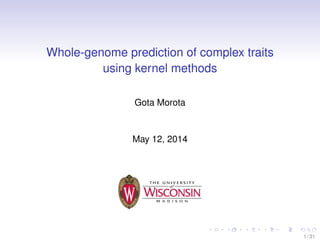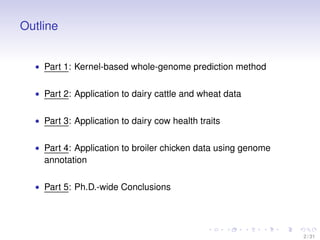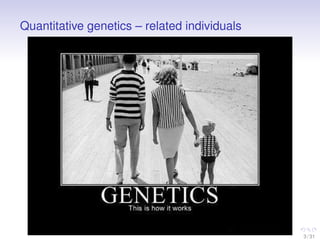Successfully reported this slideshow.

# Whole-genome prediction of complex traits using kernel methods

1

Share×
1 of 31
1 of 31

# Whole-genome prediction of complex traits using kernel methods

1

Share

Presented at my Ph.D. thesis defense. University of Wisconsin-Madison.

Presented at my Ph.D. thesis defense. University of Wisconsin-Madison.

## More Related Content

### Related Books

Free with a 14 day trial from Scribd

See all

### Related Audiobooks

Free with a 14 day trial from Scribd

See all

### Whole-genome prediction of complex traits using kernel methods

1. 1. Whole-genome prediction of complex traits using kernel methods Gota Morota May 12, 2014 1 / 31
2. 2. Outline • Part 1: Kernel-based whole-genome prediction method • Part 2: Application to dairy cattle and wheat data • Part 3: Application to dairy cow health traits • Part 4: Application to broiler chicken data using genome annotation • Part 5: Ph.D.-wide Conclusions 2 / 31
3. 3. Quantitative genetics – related individuals 3 / 31
4. 4. Prediction of complex traits from genotypes 1) GWAS-based prediction • select inﬂuential markers ﬁrst and then predict • single marker regression (2001) • marker assisted BLUP (MABLUP, 1989) 2) Whole-genome prediction • use all available markers simultaneously • Whole-genome marker regression • Kernel-based regression 4 / 31
5. 5. How to parameterize response variable y ? 1. prediction of additive genetic effects • y = E + a + 2. prediction of total genetic effects parametrically • y = E + a + d + a ∗ a + a ∗ d + d ∗ d g + 3. prediction of total genetic effects non-parametrically • y = E + g + 5 / 31
6. 6. Whole-genome marker regressions 50K SNP panel (n << p) 1. additive model • 50K RV to predict 2. additive + dominance model • 50K + 50K RV to predict! 3. additive + dominance + second-order epistasis model • 50K + 50K RV + 50K(50K-1)/2 RV to predict!! ⇓ Overparameterization!!!!! 6 / 31
7. 7. Kernel methods Regression of a phenotype on a n × n symmetric positive (semi) deﬁnite matrix K ⇓ a) Parametric • A: Pedigree kernel • G: Additive genomic kernel • D: Dominance genomic kernel b ) Non-parametric • GK: Gaussian kernel • MK: Mat´ern kernel • DK: Diffusion kernel ⇓ In non-parametric kernels, choice of the metric determines characteristics of a kernel 7 / 31
8. 8. Example of kernel methods: Genomic BLUP y = g + (where g = Xβ) (1) BLUP(ˆg) = [I + (XXT )−1 σ2 σ2 β ]−1 y. (2) Under common marker variance assumption, σ2 β = σ2 g 2 j pj(1 − pj) (3) Then, BLUP(ˆg) = [I + G−1 σ2 σ2 g ]−1 y (4) where G = XXT 2 j pj(1−pj) → Is this the best kernel?? 8 / 31
9. 9. Euclidean space – Gaussian Kernel Euclidean distance is a metric on a metric space called Euclidean space Figure 2 : 3-dimensional Euclidean space. −∞ ≤ (X, Y, Z) ≤ ∞ Suppose, we observed two individuals with 3 SNP genotypes. • ID1 = x1 = (0,2,2) • ID2 = x2 = (2,1,0) Euclidean distance (genetic distance) on R3 ||x1 − x2|| = (0 − 2)2 + (2 − 1)2 + (2 − 0)2 = 3 9 / 31
10. 10. Diffusion on 3 dimensional graph (Morota et al., (2013)) 0 1 2 012 0 1 2 1st Genotype 2ndGenotype 3rdGenotype (2,1,2) (2,0,1) (0,1,2) (0,2,0) (0,1,0) (0,1,1) (1,0,0) (2,0,0) (1,1,0) (2,1,0) (1,2,0) (2,2,0) (1,0,1) (1,1,1) (2,1,1) (0,2,1) (1,2,1) (2,2,1) (0,2,2) (1,2,2) (2,2,2) (1,0,2) (1,1,2) Figure 3 : SNP codes are viewed as coordinates of genotypes in p-dimensional space. → spatial distance 10 / 31
11. 11. Bayesian kernel ridge regression A quantitative genetics decomposition is yi = g(xi) + i (5) y − g 2 + λ g 2 H (6) • The representer theorem is used to ﬁnd the optimal g. (α) = y − Kα 2 + λ Kα 2 H (7) Here, g = Kα, is the function that minimizes (7). • Kα 2 H = α Kα, so that the function to be minimized is (α) = (y − Kα) (y − Kα) + λα Kα. (8) 11 / 31
12. 12. Kernel Averaging (Multiple Kernel Learning) • Fit three kernels simultaneously: K1, K2, and K3 K = K1 σ2 K1 ˜σ2 K + K2 σ2 K2 ˜σ2 K + K3 σ2 K3 ˜σ2 K • Example 1: Three Gaussian kernels: GK1, GK2, and GK3 K = GK1 σ2 GK1 ˜σ2 K + GK2 σ2 GK2 ˜σ2 K + GK3 σ2 GK3 ˜σ2 K • Example 2: Three parametric kernels: G, D, and G#D K = G σ2 G ˜σ2 K + D σ2 D ˜σ2 K + (G#D) σ2 GD ˜σ2 K 12 / 31
13. 13. Part 2: Application (Morota et al., 2013) Holstein • 7,902 Holstein bulls (USDA-ARS AIPL) • 43,382 SNPs • PTA of productive life (PL) Wheat • 599 inbred lines • 1,279 binary markers • average grain yield Methods • Bayesian kernel ridge regression (RKHS method) • Cross-validation 13 / 31
14. 14. Averages of kernel elements and their predictive correlations for the Holstein data Kernel θ k(xi, xj) Cor(ˆytest , yPTA ) DK 10 0.138 0.727 11 0.483 0.745 11.5 0.644 0.739 12 0.765 0.739 13 0.907 0.734 14 0.966 0.729 GK 5 × 10−5 0.237 0.721 2 × 10−5 0.551 0.736 1 × 10−5 0.749 0.742 5 × 10−6 0.866 0.736 3 × 10−6 0.917 0.734 1 × 10−6 0.971 0.729 G1 NA -0.000126 0.729 G2 NA -0.000113 0.730 14 / 31
15. 15. Averages of kernel elements and their predictive correlations for the wheat data Kernel θ k(xi, xj) Cor(ˆytest , ytrain ) Diffusion 3 0.136 0.586 3.25 0.289 0.580 3.5 0.466 0.577 4 0.752 0.547 5 0.962 0.522 Gaussian 0.005 0.134 0.582 0.003 0.290 0.579 0.002 0.434 0.562 0.001 0.655 0.558 0.0005 0.809 0.556 G1 NA -0.003 0.518 G2 NA -0.003 0.521 15 / 31
16. 16. Part 3: Application (Morota et al., 2014) Data • 4,482 dairy cows (Zoetis) • 41,266 SNPs • EBV and PCP of six health traits Two steps approach 1. variance components estimation using parametric kernels 2. predict total genetic values using parametric and non-parametric kernels Methods • Bayesian kernel ridge regression (RKHS method) • Cross-validation 16 / 31
17. 17. Estimated ratios of variance components (weights) Traits Types Variance Components VG/VP VD/VP VGD/VP KET PCP 0.09 0.13 0.14 EBV 0.25 0.03 0.01 DA PCP 0.06 0.09 0.25 EBV 0.39 0.04 0.30 RP PCP 0.05 0.09 0.35 EBV 0.27 0.03 0.07 LAME PCP 0.06 0.07 0.39 EBV 0.39 0.03 0.27 METR PCP 0.06 0.07 0.21 EBV 0.31 0.05 0.42 CM PCP 0.06 0.07 0.26 EBV 0.36 0.02 0.16 17 / 31